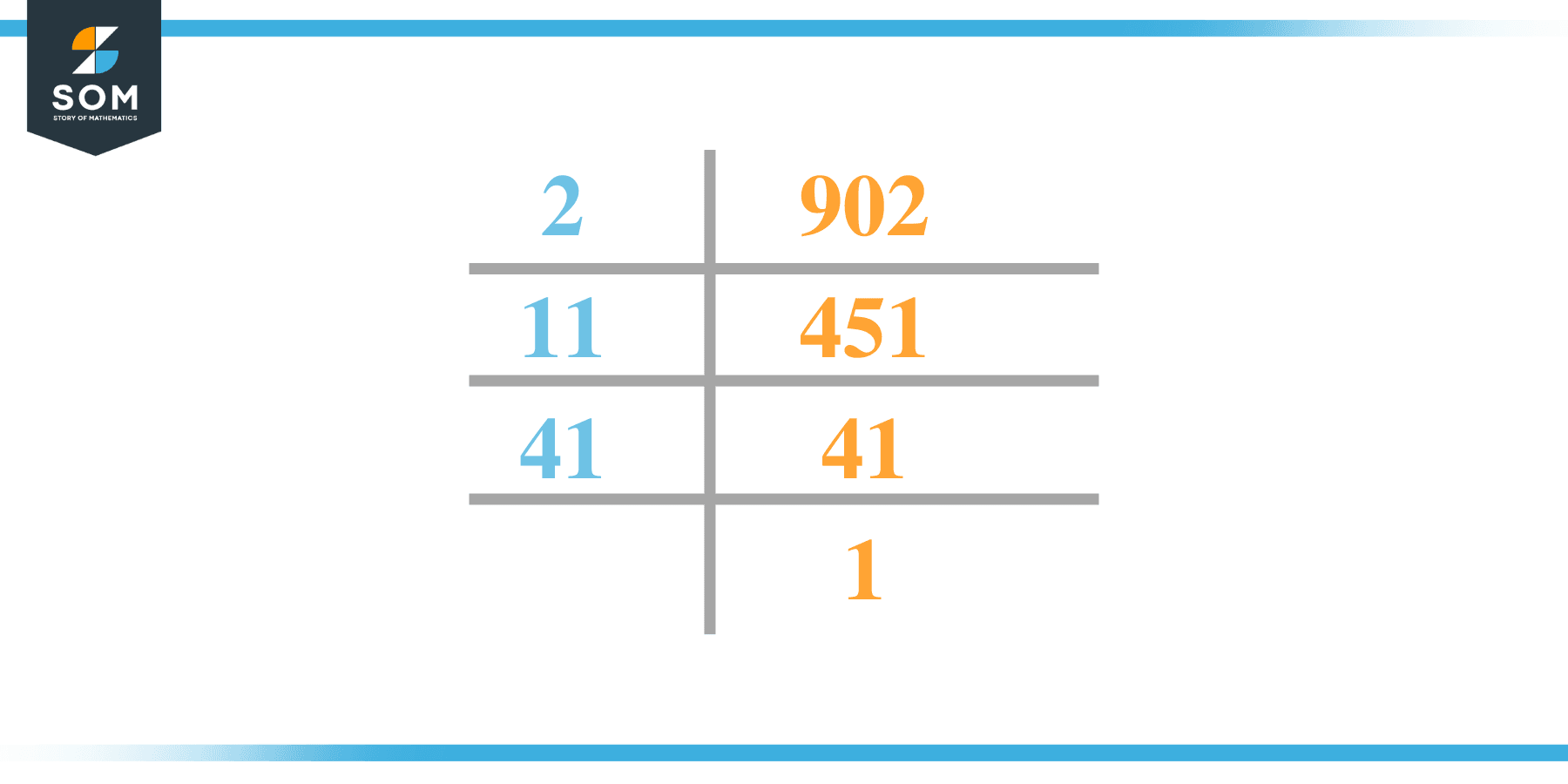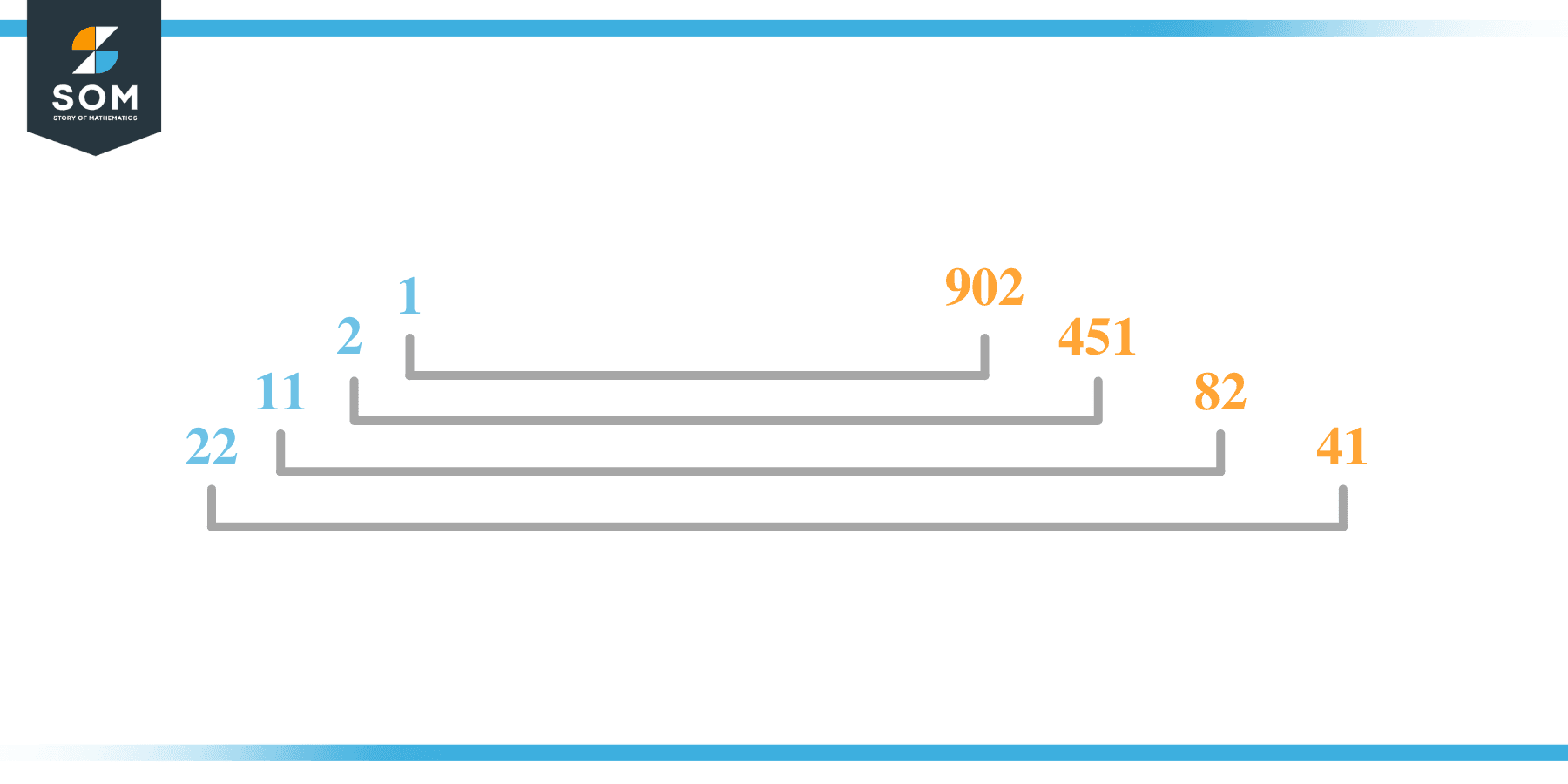# Factors of 902: Prime Factorization, Methods, and Examples

The factors of 902 are the numbers that can be evenly divided into 902. There are 8 such numbers that exist on the number line that can be evenly divided into 902.It has no square factors meaning, there is no such number that is when multiplied by itself, the answer is 902.

### Factors of 902

Here are the factors of number 902.

Factors of 902: 1, 2, 11, 22, 41, 82, 451, and 902

### Negative Factors of 902

The negative factors of 902 are similar to its positive aspects, just with a negative sign.

Negative Factors of 902: -1, -2, -11, -22, -41, -82, -451, and -902

### Prime Factorization of 902

The prime factorization of 902 is the way of expressing its prime factors in the product form.

Prime Factorization: 2 x 11 x 41

In this article, we will learn about the factors of 902 and how to find them using various techniques such as upside-down division, prime factorization, and factor tree.

## What Are the Factors of 902?

The factors of 902 are 1, 2, 11, 22, 41, 82, 451, and 902 . These numbers are the factors as they do not leave any remainder when divided by 902.

The factors of 902 are classified as prime numbers and composite numbers. The prime factors of the number 902 can be determined using the prime factorization technique.

## How To Find the Factors of 902?

You can find the factors of 902 by using the rules of divisibility. The divisibility rule states that any number, when divided by any other natural number, is said to be divisible by the number if the quotient is the whole number and the resulting remainder is zero.

To find the factors of 902, create a list containing the numbers that are exactly divisible by 902 with zero remainders. One important thing to note is that 1 and 902 are the 902’s factors as every natural number has 1 and the number itself as its factor.

1 is also called the universal factor of every number. The factors of 902 are determined as follows:

$\dfrac{902}{1} = 902$

$\dfrac{902}{2} = 451$

$\dfrac{902}{11} = 82$

$\dfrac{902}{22} = 41$

$\dfrac{902}{41} = 22$

$\dfrac{902}{82} = 11$

$\dfrac{902}{451} = 2$

$\dfrac{902}{902} = 1$

Therefore, 1, 2, 11, 22, 41, 82, 451, and 902 are the factors of 902.

### Total Number of Factors of 902

For 902, there are 8 positive factors and 8 negative ones. So in total, there are 16 factors of 902.

To find the total number of factors of the given number, follow the procedure mentioned below:

1. Find the factorization/prime factorization of the given number.
2. Demonstrate the prime factorization of the number in the form of exponent form.
3. Add 1 to each of the exponents of the prime factor.
4. Now, multiply the resulting exponents together. This obtained product is equivalent to the total number of factors of the given number.

By following this procedure, the total number of factors of 902 is given as:

Factorization of 902 is 1 x 2 x 11 x 41.

The exponent of 1, 2, 11, and 41 is 1.

Adding 1 to each and multiplying them together results in 16.

Therefore, the total number of factors of 902 is 16. 8 is positive, and 8 factors are negative.

### Important Notes

Here are some essential points that must be considered while finding the factors of any given number:

• The factor of any given number must be a whole number.
• The factors of the number cannot be in the form of decimals or fractions.
• Factors can be positive as well as negative.
• Negative factors are the additive inverse of the positive factors of a given number.
• The factor of a number cannot be greater than that number.
• Every even number has 2 as its prime factor, the smallest prime factor.

## Factors of 902 by Prime FactorizationThe number 902 is a composite number. Prime factorization is a valuable technique for finding the number’s prime factors and expressing the number as the product of its prime factors.

Before finding the factors of 902 using prime factorization, let us find out what prime factors are. Prime factors are the factors of any given number that are only divisible by 1 and themselves.

To start the prime factorization of 902, start dividing by its most minor prime factor. First, determine that the given number is either even or odd. If it is an even number, then 2 will be the smallest prime factor.

Continue splitting the quotient obtained until 1 is received as the quotient. The prime factorization of 902 can be expressed as:

902 = 2 x 11 x 41

## Factors of 902 in PairsThe factor pairs are the duplet of numbers that, when multiplied together, result in the factorized number. Factor pairs can be more than one depending on the total number of factors given.

For 902, the factor pairs can be found as:

1 x 902 = 902

2 x 451 = 902

11 x 82 = 902

22 x 41 = 902

The possible factor pairs of 902 are given as (1, 902), (2, 451), (11, 82), and (22, 41).

All these numbers in pairs, when multiplied, give 902 as the product.

The negative factor pairs of 902 are given as:

-1 x -902 = 902

-2 x -451 = 902

-11 x -82 = 902

-22 x -41 = 902

It is important to note that in negative factor pairs, the minus sign has been multiplied by the minus sign, due to which the resulting product is the original positive number. Therefore, -1, -2, -11, -22, -41, -82, -451, and -902 are called negative factors of 902.

The list of all the factors of 902, including positive as well as negative numbers, is given below.

Factor list of 902: 1, 2, 11, 22, 41, 82, 451, 902

## Factors of 902 Solved Examples

To better understand the concept of factors, let’s solve some examples.

### Example 1

How many factors of 902 are there?

### Solution

The total number of Factors of 892 is m8

Factors of 902 are 1, 2, 11, 22, 41, 82, 451, and 902 .

### Example 2

Find the factors of 902 using prime factorization.

### Solution

The prime factorization of 902 is given as:

902 $\div$ 2 = 451

451 $\div$ 211 = 41

41 $\div$ 41 = 1

So the prime factorization of 902 can be written as:

2 x 11 x 41 = 902